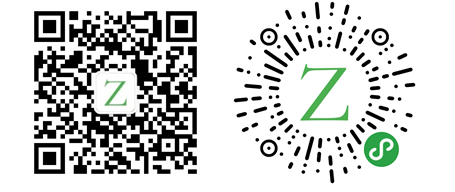# 走进 Rust：参考和借阅

Rust 大约 6762 字

## 参考和借阅

``````fn main() {
let s1 = String::from("hello");

let len = calculate_length(&s1);

println!("The length of '{}' is {}.", s1, len);
}

fn calculate_length(s: &String) -> usize {
s.len()
}````````````let s1 = String::from("hello");

let len = calculate_length(&s1);``````

``````fn calculate_length(s: &String) -> usize { // s 是 String 类型的引用
s.len()
} // 这一行，s 超出作用域。但因为 s 没有拥有它引用的对象的所有权，所以啥事情都不会发生（即不发生 drop ）``````

``````fn main() {
let s = String::from("hello");

change(&s);
}

fn change(some_string: &String) {
some_string.push_str(", world");
}``````

``````\$ cargo run
Compiling ownership v0.1.0 (file:///projects/ownership)
error[E0596]: cannot borrow `*some_string` as mutable, as it is behind a `&` reference
--> src/main.rs:8:5
|
7 | fn change(some_string: &String) {
|                        ------- help: consider changing this to be a mutable reference: `&mut std::string::String`
8 |     some_string.push_str(", world");
|     ^^^^^^^^^^^ `some_string` is a `&` reference, so the data it refers to cannot be borrowed as mutable

error: aborting due to previous error

error: could not compile `ownership`.

To learn more, run the command again with --verbose.``````

## 可变引用

``````fn main() {
let mut s = String::from("hello");

change(&mut s);
}

fn change(some_string: &mut String) {
some_string.push_str(", world");
}``````

``````fn main() {
let mut s = String::from("hello");

let r1 = &mut s;
let r2 = &mut s;

println!("{}, {}", r1, r2);
}``````

``````\$ cargo run
Compiling ownership v0.1.0 (file:///projects/ownership)
error[E0499]: cannot borrow `s` as mutable more than once at a time
--> src/main.rs:5:14
|
4 |     let r1 = &mut s;
|              ------ first mutable borrow occurs here
5 |     let r2 = &mut s;
|              ^^^^^^ second mutable borrow occurs here
6 |
7 |     println!("{}, {}", r1, r2);
|                        -- first borrow later used here

error: aborting due to previous error

error: could not compile `ownership`.

To learn more, run the command again with --verbose.``````

• 两个或多个指针同时访问相同的数据。
• 至少有一个指针用于写入数据。
• 没有被用于访问数据时上锁的机制。

``````fn main() {
let mut s = String::from("hello");

{
let r1 = &mut s;
} // r1 在这一行超出作用域，所以我们可以创建一个新的引用

let r2 = &mut s;
}``````

``````fn main() {
let mut s = String::from("hello");

let r1 = &s; // 没问题
let r2 = &s; // 没问题
let r3 = &mut s; // 大问题

println!("{}, {}, and {}", r1, r2, r3);
}``````

``````\$ cargo run
Compiling ownership v0.1.0 (file:///projects/ownership)
error[E0502]: cannot borrow `s` as mutable because it is also borrowed as immutable
--> src/main.rs:6:14
|
4 |     let r1 = &s; // no problem
|              -- immutable borrow occurs here
5 |     let r2 = &s; // no problem
6 |     let r3 = &mut s; // BIG PROBLEM
|              ^^^^^^ mutable borrow occurs here
7 |
8 |     println!("{}, {}, and {}", r1, r2, r3);
|                                -- immutable borrow later used here

error: aborting due to previous error

error: could not compile `ownership`.

To learn more, run the command again with --verbose.``````

``````fn main() {
let mut s = String::from("hello");

let r1 = &s; // 没问题
let r2 = &s; // 没问题
println!("{} and {}", r1, r2);
// r1 和 r2 在这之后不再使用

let r3 = &mut s; // 没问题
println!("{}", r3);
}``````

## 悬空引用（空指针）

``````fn main() {
let reference_to_nothing = dangle();
}

fn dangle() -> &String {
let s = String::from("hello");

&s
}``````

``````\$ cargo run
Compiling ownership v0.1.0 (file:///projects/ownership)
error[E0106]: missing lifetime specifier
--> src/main.rs:5:16
|
5 | fn dangle() -> &String {
|                ^ help: consider giving it a 'static lifetime: `&'static`
|
= help: this function's return type contains a borrowed value, but there is no value for it to be borrowed from

error: aborting due to previous error

error: could not compile `ownership`.

To learn more, run the command again with --verbose.``````

``````this function's return type contains a borrowed value, but there is no value
for it to be borrowed from.``````

``````fn dangle() -> &String { // dangle 函数返回一个 String 类型的引用

let s = String::from("hello"); // s 是一个新的 String 类型变量

&s // 我们返回一个 String 类型的引用 s
} // 这一步，s 超出作用域并且并销毁。它的内存释放了。
// 报错!``````

``````fn main() {
let string = no_dangle();
}

fn no_dangle() -> String {
let s = String::from("hello");

s
}``````

## 引用的规则

• 在任何给定时间，你都可以声明一个可变引用或任意数量的不可变引用。
• 引用必须始终有效。

————        END        ————

Give me a Star, Thanks:)

https://github.com/fendoudebb